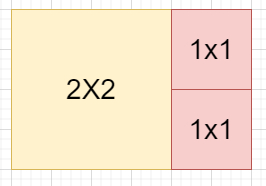Tiling a Rectangle with the Fewest Squares in C++

C++Server Side ProgrammingProgramming

Suppose we have a rectangle of size n x m. We have to find the minimum number of integers sided square objects that can tile the rectangles.

So, if the input is like n = 2 and m = 3,then the output will be 3, as we need three blocks.

To solve this, we will follow these steps −

• Define one map m

• res := inf

• Define a function dfs(), this will take n, m, an array h, cnt,

• if cnt >= res, then −

• return

• isFull := true

• pos := -1, minH := inf

• for initialize i := 1, when i <= n, update (increase i by 1), do −

• if h[i] < m, then −

• isFull := false

• if h[i] < minH, then −

• minH := h[i]

• pos := i

• if isFull is non-zero, then −

• res := minimum of res and cnt

• return

• key := 0

• base := m + 1

• for initialize i := 1, when i <= n, update (increase i by 1), do −

• key := key + h[i] * base

• base := base * (m + 1)

• if key is in s and s[key] <= cnt, then −

• return

• s[key] := cnt

• end := pos

• while (end + 1 <= n and h[end + 1] is same as h[pos] and (end + 1 - pos + 1 + minH)

• <= m), do −

• (increase end by 1)

• for initialize j := end, when j >= pos, update (decrease j by 1), do −

• curH := j - pos + 1

• Define an array next of size n + 1

• for initialize i := 1, when i <= n, update (increase i by 1), do −

• next[i] := h[i]

• for initialize k := pos, when k <= j, update (increase k by 1), do −

• next[k] := next[k] + curH

• dfs(n, m, next, cnt + 1)

• From the main method do the following −

• if n is same as m, then −

• return 1

• if n > m, then

• swap(n, m)

• Define an array h of size n + 1

• dfs(n, m, h, 0)

• return res

Let us see the following implementation to get better understanding −

Example

Live Demo

#include <bits/stdc++.h>
using namespace std;
class Solution {
public:
map<int, int> s;
int res = INT_MAX;
void dfs(int n, int m, vector<int> h, int cnt){
if (cnt >= res)
return;
bool isFull = true;
int pos = -1, minH = INT_MAX;
for (int i = 1; i <= n; i++) {
if (h[i] < m)
isFull = false;
if (h[i] < minH) {
minH = h[i];
pos = i;
}
}
if (isFull) {
res = min(res, cnt);
return;
}
long key = 0;
long base = m + 1;
for (int i = 1; i <= n; i++) {
key += h[i] * base;
base *= m + 1;
}
if (s.find(key) != s.end() && s[key] <= cnt)
return;
s[key] = cnt;
int end = pos;
while (end + 1 <= n && h[end + 1] == h[pos] && (end + 1 - pos + 1 + minH) <= m)
end++;
for (int j = end; j >= pos; j--) {
int curH = j - pos + 1;
vector<int> next(n + 1);
for (int i = 1; i <= n; i++)
next[i] = h[i];
for (int k = pos; k <= j; k++) {
next[k] += curH;
}
dfs(n, m, next, cnt + 1);
}
}
int tilingRectangle(int n, int m){
if (n == m)
return 1;
if (n > m)
swap(n, m);
vector<int> h(n + 1);
dfs(n, m, h, 0);
return res;
}
};
main(){
Solution ob;
cout << (ob.tilingRectangle(2, 3));
}

2,3

3